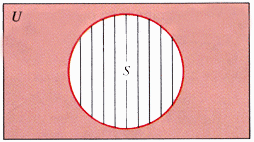# Section 2.1: Sets, Subsets, and Venn Diagrams

## 2.1 Outline

1. Denoting sets
1. undefined term; set
2. circular definition
3. specifying sets
1. description method
2. roster method
4. well-defined set
5. terms
1. members
2. elements
3. belong to
4. contained in
6. Sets of numbers
1. natural numbers
2. counting numbers
3. integers
4. rational numbers
7. set-builder notation
2. Equal and equivalent sets
1. equal sets
2. equivalent sets
3. cardinality of a set
4. cardinal number
3. Universal and empty sets
1. definitions
2. notation for
4. Venn diagrams
1. complement
2. general representation of a set
5. Subsets and proper subsets
1. definitions
2. disjoint sets
3. improper subset
4. number of subsets
5. general representation of two sets
6. general representation of three sets

## 2.1 Essential Ideas

Denoting Sets

Sets are defined using the description or roster methods.
The objects in a set are called members or elements of the set.
The cardinality of a set is the number of elements in a set.
Two sets are equal if they contain the same number.
Two sets are equivalent if they have the same number of elements.

Sets of Numbers

Natural Numbers: {1, 2, 3, … }
Whole Numbers: {0, 1, 2, 3, … }
Integers: {…,, -2, -1, 0, 1, 2, … }
Rational Numbers: {a/b where a is an integer and b a nonzero integer}

Special Sets

The universal set contains all the elements under consideration in a given discussion.
The empty set contains no elements.

Venn Diagrams

One set divides the universe into 2 regions.
Two sets divide the universe into 4 regions.
Three sets divide the universe into 8 regions.

ComplementThe complement of a set S  is consists of everything that is not in S.

Subsets

A set A is a subset of a set B, if every element of A is also an element of B.

A set A is a proper subset of a set B, if every element of A is also an element of B and A and B are not equal sets.